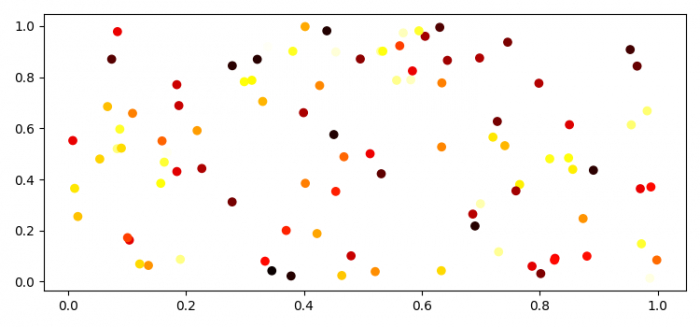# Scatter a 2D numpy array in matplotlib

MatplotlibPythonData Visualization

#### Python Data Science basics with Numpy, Pandas and Matplotlib

Most Popular

63 Lectures 6 hours

#### Data Visualization using MatPlotLib & Seaborn

11 Lectures 4 hours

#### MatPlotLib with Python

9 Lectures 2.5 hours

To scatter a 2D numpy array in matplotlib, we can take the following steps −

## Steps

• Set the figure size and adjust the padding between and around the subplots.

• Create random data of 100×3 dimension.

• Use the scatter() method to plot 2D numpy array, i.e., data.

• To display the figure, use show() method.

## Example

import numpy as np
from matplotlib import pyplot as plt

# Set the figure size
plt.rcParams["figure.figsize"] = [7.50, 3.50]
plt.rcParams["figure.autolayout"] = True

# Random data of 100×3 dimension
data = np.array(np.random.random((100, 3)))

# Scatter plot
plt.scatter(data[:, 0], data[:, 1], c=data[:, 2], cmap='hot')

# Display the plot
plt.show()

## Output

It will produce the following output −